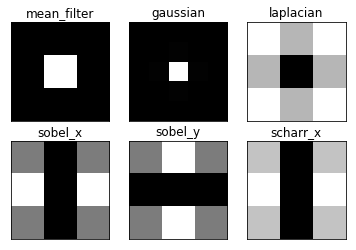# 6.1. 傅里叶变换¶

## 6.1.1. 目标¶

-要使用OpenCV查找图像的傅里叶变换-要利用Numpy中可用的FFT函数-傅里叶变换的一些应用-我们将看到以下函数： cv2.dft（）cv2.idft（）

## 6.1.3. 傅里叶变换¶

>>> %matplotlib inline
>>> import cv2
>>> import numpy as np
>>> from matplotlib import pyplot as plt
>>>
>>> f = np.fft.fft2(img)
>>> fshift = np.fft.fftshift(f)
>>> magnitude_spectrum = 20*np.log(np.abs(fshift))
>>>
>>> plt.subplot(121),plt.imshow(img, cmap = 'gray')
>>> plt.title('Input Image'), plt.xticks([]), plt.yticks([])
>>> plt.subplot(122),plt.imshow(magnitude_spectrum, cmap = 'gray')
>>> plt.title('Magnitude Spectrum'), plt.xticks([]), plt.yticks([])
>>> plt.show()>>> rows, cols = img.shape
>>> crow,ccol = int(rows/2) , int(cols/2)

>>> crow

171

>>> ccol

274

>>> fshift

array([[ -124.           +0.j        ,   -42.19440414 -877.03617166j,
223.27548059+1046.60850634j, ...,
1202.61938833 +206.61865249j,   223.27548059-1046.60850634j,
-42.19440414 +877.03617166j],
[  208.74536514  +64.60669315j,  1002.28500993 -234.50419799j,
1506.32632304+1222.65769814j, ...,
-1389.57648774 -150.87464627j,   109.36759417 +488.69796424j,
-57.03604421 +606.24574085j],
[  900.36223857 +317.76577457j,   653.10253934 -411.72777201j,
-1280.58956522+1186.46570157j, ...,
-121.90161098+1372.27974992j,   519.73405635 +156.14284965j,
-549.90306456 -294.50881028j],
...,
[  222.83408186 -992.83030536j,    12.48466653+1024.31486962j,
627.72474919 -709.13462585j, ...,
1305.90353042 +971.79340223j, -1576.22476438-1240.03645033j,
768.60806672+1088.72533153j],
[  900.36223857 -317.76577457j,  -549.90306456 +294.50881028j,
519.73405635 -156.14284965j, ...,
461.36292583+1732.90045983j, -1280.58956522-1186.46570157j,
653.10253934 +411.72777201j],
[  208.74536514  -64.60669315j,   -57.03604421 -606.24574085j,
109.36759417 -488.69796424j, ...,
-1478.63347024+1111.98404021j,  1506.32632304-1222.65769814j,
1002.28500993 +234.50419799j]])

>>> fshift[crow-30:crow+30, ccol-30:ccol+30] = 0

>>> f_ishift = np.fft.ifftshift(fshift)
>>> img_back = np.fft.ifft2(f_ishift)
>>> img_back = np.abs(img_back)
>>>
>>> plt.subplot(131),plt.imshow(img, cmap = 'gray')
>>> plt.title('Input Image'), plt.xticks([]), plt.yticks([])
>>> plt.subplot(132),plt.imshow(img_back, cmap = 'gray')
>>> plt.title('Image after HPF'), plt.xticks([]), plt.yticks([])
>>> plt.subplot(133),plt.imshow(img_back)
>>> plt.title('Result in JET'), plt.xticks([]), plt.yticks([])
>>>
>>> plt.show()## 6.1.4. OpenCV中的傅里叶变换¶

OpenCV提供了 cv2.dft（）cv2.idft（） 为了这个。它返回与前面相同的结果，但有两个通道。第一个通道将具有结果的实部，第二个通道将具有结果的虚部。输入图像应首先转换为np.float32。我们看看怎么做。

>>> import numpy as np
>>> import cv2
>>> from matplotlib import pyplot as plt
>>>
>>>
>>> dft = cv2.dft(np.float32(img),flags = cv2.DFT_COMPLEX_OUTPUT)
>>> dft_shift = np.fft.fftshift(dft)
>>>
>>> magnitude_spectrum = 20*np.log(cv2.magnitude(dft_shift[:,:,0],dft_shift[:,:,1]))
>>>
>>> plt.subplot(121),plt.imshow(img, cmap = 'gray')
>>> plt.title('Input Image'), plt.xticks([]), plt.yticks([])
>>> plt.subplot(122),plt.imshow(magnitude_spectrum, cmap = 'gray')
>>> plt.title('Magnitude Spectrum'), plt.xticks([]), plt.yticks([])
>>> plt.show()>>> rows, cols = img.shape
>>> crow,ccol = int(rows/2) , int(cols/2)
>>>
>>> # create a mask first, center square is 1, remaining all zeros
>>>
>>> # apply mask and inverse DFT
>>> f_ishift = np.fft.ifftshift(fshift)
>>> img_back = cv2.idft(f_ishift)
>>> img_back = cv2.magnitude(img_back[:,:,0],img_back[:,:,1])
>>>
>>> plt.subplot(121),plt.imshow(img, cmap = 'gray')
>>> plt.title('Input Image'), plt.xticks([]), plt.yticks([])
>>> plt.subplot(122),plt.imshow(img_back, cmap = 'gray')
>>> plt.title('Magnitude Spectrum'), plt.xticks([]), plt.yticks([])
>>> plt.show()## 6.1.5. DFT的性能优化¶

>>> img = cv2.imread('/cvdata/messi5.jpg',0)
>>> rows,cols = img.shape
>>> rows,cols

(342, 548)

>>> nrows = cv2.getOptimalDFTSize(rows)
>>> ncols = cv2.getOptimalDFTSize(cols)

>>> nrows, ncols

(360, 576)


>>> nimg = np.zeros((nrows,ncols))
>>> nimg[:rows,:cols] = img


>>> right = ncols - cols
>>> bottom = nrows - rows
>>> bordertype = cv2.BORDER_CONSTANT #just to avoid line breakup in PDF file
>>> nimg = cv2.copyMakeBorder(img,0,bottom,0,right,bordertype, value = 0)


>>> %timeit fft1 = np.fft.fft2(img)

10 loops, best of 5: 31.4 ms per loop

>>> # 10 loops, best of 3: 40.9 ms per loop
>>>

>>> %timeit fft2 = np.fft.fft2(img,[nrows,ncols])

100 loops, best of 5: 10.1 ms per loop

>>>
>>> # 100 loops, best of 3: 10.4 ms per loop


>>> %timeit dft1= cv2.dft(np.float32(img),flags=cv2.DFT_COMPLEX_OUTPUT)
>>> # 100 loops, best of 3: 13.5 ms per loop

100 loops, best of 5: 11.6 ms per loop

>>>
>>> %timeit dft2= cv2.dft(np.float32(nimg),flags=cv2.DFT_COMPLEX_OUTPUT)
>>> # 100 loops, best of 3: 3.11 ms per loop

100 loops, best of 5: 2.55 ms per loop


## 6.1.6. 为什么拉普拉斯是高通滤波器？¶

>>> import cv2
>>> import numpy as np
>>> from matplotlib import pyplot as plt
>>>
>>> # simple averaging filter without scaling parameter
>>> mean_filter = np.ones((3,3))
>>>
>>> # creating a guassian filter
>>> x = cv2.getGaussianKernel(5,10)
>>> gaussian = x*x.T
>>>
>>> # different edge detecting filters
>>> # scharr in x-direction
>>> scharr = np.array([[-3, 0, 3],
>>>                    [-10,0,10],
>>>                    [-3, 0, 3]])
>>> # sobel in x direction
>>> sobel_x= np.array([[-1, 0, 1],
>>>                    [-2, 0, 2],
>>>                    [-1, 0, 1]])
>>> # sobel in y direction
>>> sobel_y= np.array([[-1,-2,-1],
>>>                    [0, 0, 0],
>>>                    [1, 2, 1]])
>>> # laplacian
>>> laplacian=np.array([[0, 1, 0],
>>>                     [1,-4, 1],
>>>                     [0, 1, 0]])
>>>
>>> filters = [mean_filter, gaussian, laplacian, sobel_x, sobel_y, scharr]
>>> filter_name = ['mean_filter', 'gaussian','laplacian', 'sobel_x', \
>>>                 'sobel_y', 'scharr_x']
>>> fft_filters = [np.fft.fft2(x) for x in filters]
>>> fft_shift = [np.fft.fftshift(y) for y in fft_filters]
>>> mag_spectrum = [np.log(np.abs(z)+1) for z in fft_shift]
>>>
>>> for i in range(6):
>>>     plt.subplot(2,3,i+1),plt.imshow(mag_spectrum[i],cmap = 'gray')
>>>     plt.title(filter_name[i]), plt.xticks([]), plt.yticks([])
>>>
>>> plt.show()